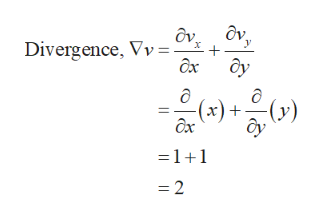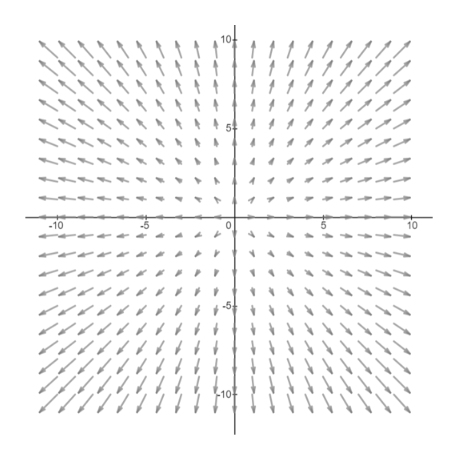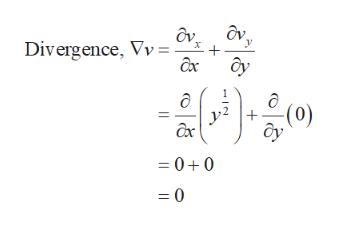For the given 2D flow fields:Find the divergenceSketch the velocity fielda) v(x,y)=(vx(x,y), vy(x,y))=(x,y)b) v(x,y)=(vx(x,y), vy(x,y))= (y1/2,0)c) v(x,y)=(vx(x,y), vy(x,y)= (-y,x)

Question

For the given 2D flow fields:

• Find the divergence
• Sketch the velocity field

a) v(x,y)=(vx(x,y), vy(x,y))=(x,y)

b) v(x,y)=(vx(x,y), vy(x,y))= (y1/2,0)

c) v(x,y)=(vx(x,y), vy(x,y)= (-y,x)

Step 1

To find the divergence and sketch the velocity field of the following 2D flow fields.

(a) v(x,y)=(vx(x,y), vy(x,y))=(x,y)help_outlineImage TranscriptioncloseDivergence, Vv=, ax ôy (x)+y) =1+1 = 2 fullscreen
Step 2

The velocity field is as follows.help_outlineImage Transcriptionclose10 10 -10 5 0 5 -10 fullscreen
Step 3

(b) v(x,y)=(vx(x,y), vy(x,y))...help_outlineImage TranscriptioncloseDivergence, Vv=- ax (0) + ax =00 =0 fullscreen

Want to see the full answer?

See Solution

Want to see this answer and more?

Our solutions are written by experts, many with advanced degrees, and available 24/7

See Solution
Tagged in

Calculus